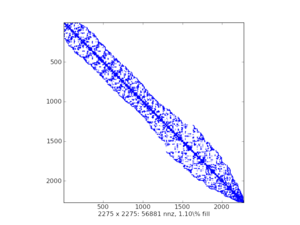# 2.5.1. Introduction¶

(dense) matrix is:

• mathematical object
• data structure for storing a 2D array of values

important features:

• memory allocated once for all items
• usually a contiguous chunk, think NumPy ndarray

## 2.5.1.1. Why Sparse Matrices?¶

• the memory, that grows like n**2

• small example (double precision matrix):

```>>> import numpy as np
>>> import matplotlib.pyplot as plt
>>> x = np.linspace(0, 1e6, 10)
>>> plt.plot(x, 8.0 * (x**2) / 1e6, lw=5)
[<matplotlib.lines.Line2D object at ...>]
>>> plt.xlabel('size n')
Text(...'size n')
>>> plt.ylabel('memory [MB]')
Text(...'memory [MB]')
```

## 2.5.1.2. Sparse Matrices vs. Sparse Matrix Storage Schemes¶

• sparse matrix is a matrix, which is almost empty
• storing all the zeros is wasteful -> store only nonzero items
• think compression
• pros: huge memory savings
• cons: depends on actual storage scheme, (*) usually does not hold

## 2.5.1.3. Typical Applications¶

• solution of partial differential equations (PDEs)
• the finite element method
• mechanical engineering, electrotechnics, physics, …
• graph theory
• nonzero at (i, j) means that node i is connected to node j
• natural language processing
• nonzero at (i, j) means that the document i contains the word j

## 2.5.1.5. Sparsity Structure Visualization¶

• `spy()` from `matplotlib`
• example plots: